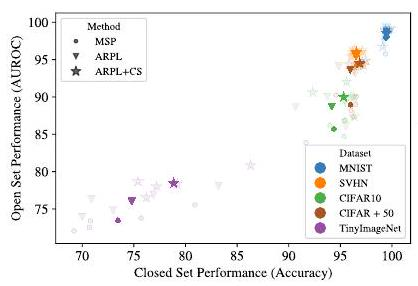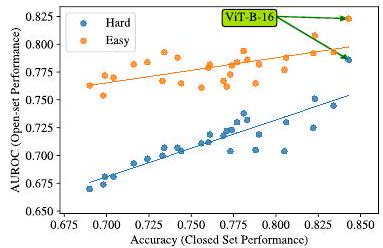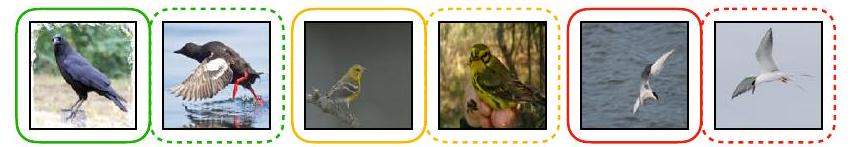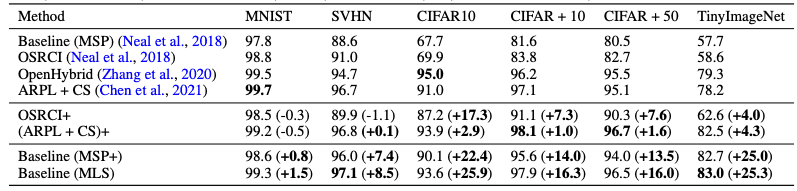# Catch up on the latest AI articles# Proposal For An Out-of-distribution Detection Method And A New Benchmark That Allows Models To Identify

3 main points
✔️ Out-of-distribution (OoD, out-of-distribution) detection is the identification of data that is not in the training dataset
✔️ Good models that can classify a limited number of classes will perform in OoD detection
✔️ Successfully detect novelty in data by devising loss functions

written by Sagar VazeKai HanAndrea VedaldiAndrew Zisserman
(Submitted on 12 Oct 2021 (v1), last revised 13 Apr 2022 (this version, v2)])

Subjects:  Computer Vision and Pattern Recognition (cs.CV); Machine Learning (cs.LG)code：The images used in this article are from the paper, the introductory slides, or were created based on them.

## summary

In the real world, there are countless objects such as animals, etc. Therefore, the classifier can learn only a small fraction of the classes in reality, but the classifier tries to fit samples that are not in the trained classes into the known classes. This means that no matter how good the model is, the classification itself will be wrong, so this paper introduces a method to make the classifier identify "this is not in the trained dataset". This is the reason why we introduce a method to make the classifier identify "This is not included in the trained dataset.

In this paper, we propose a metric called Semantic Shift Benchmark (SSB), which successfully detects the semantic (semantic) novelty of a sample.

## first of all

Deep learning has done well in image recognition tasks on closed sets with a limited number of classification classes; the next challenge is open-set recognition (OSR), i.e., the model needs to determine whether a given data set is part of the training dataset. recognition (OSR) on open-set datasets, i.e., the model needs to determine whether a given data set is part of the training dataset or not.

The OSR problem was formalized by Scheirer et al. and has been worked on by several excellent researchers since then, and the baseline for evaluating the OSR problem is a model trained on a closed data set. The model is trained using the cross-entropy error as the loss function and the output is a softmax function; henceforth this approach will be referred to simply as the baseline or maximum It should be noted that existing reports of OSR using MNIST and TinyImageNet have significantly outperformed the baseline. The baseline is called the

Interestingly, however, the results show that there is a correlation between the performance of the closed-set and open-set models.

## related research

Scheirer et al. formulated the OSR problem; Bendale and Boult proposed the OpenMax approach based on Extreme Value Theory (EVT); Neal et al. worked on OSR using images generated by a Generative Adversarial Network (GAN), which later led to the creation of the OSR dataset.

OSR is closely related to out-of-distribution detection (OoD detection), novelty detection, and anomaly In this paper, we propose a new benchmark to compare OSR and OoD detection. Therefore, in this paper, we distinguish OSR from OoD detection by proposing a new benchmark and presenting a novelty in this field.

### Correlating performance on closed and open sets

It is thought that a classifier that performs well on a data set with a limited number of classes (henceforth referred to as a closed set) will paradoxically perform poorly on a data set with an unlimited number of classes (henceforth referred to as an open set). However, in this paper, we show that the performance on the closed set correlates well with the performance on the open set. set correlates with the performance on the open set.

\begin{equation}\mathcal{D}_{\text {train }}=\\left\{\left(\mathbf{x}_{i}, y_{i}\right)\right\}_{i=1}^{N} \subset \mathcal{X} \mathcal{C}\end{equation}

Let us first formulate the OSR: the training data D_train is contained in the product of the input space Χ (note: capital chi) and the number of known classes C.

$$\mathcal{D}_{\text {test-closed }}=\left\{\left(\mathbf{x}_{i}, y_{i}\right)\right\}_{i=1}^{M} \subset \mathcal{X} \times \mathcal{C}$$

The validation dataset can be represented in the same way as above; this is the closed set setup.

$$\mathcal{D}_{\text {test-open }}=\left\{\left(\mathbf{x}_{i}, y_{i}\right)\right\}_{i=1}^{M^{\prime}} \subset \mathcal{X} \times(\mathcal{C} \cup \mathcal{U})$$

In the open set, there is no limit to the number of classes, so the number of elements increases by the unknown class U as well as the number of known classes C.

$$p(y \mid \mathbf{x})$$

For a closed set, the probability distribution p of being in that class can be expressed as above, but for an open set, we also need the probability of being in a known class C or not.

$$p(y \mid \mathbf{x}, y \in \mathcal{C})$$

$$\mathcal{S}(y \in \mathcal{C} \mathbf{x})$$

Therefore, a condition is attached to the probability p. S is the score for inclusion in the known class C or not.Now, the above is a correlation chart between the performance on the closed set (Accuracy) and the performance on the open set (AUROC).In this paper, we use a lightweight model of VGG16 (VGG32) and existing datasets (MNIST, SVHN, CIFAR, etc.) are used for evaluation; the OSR benchmarks are MSP (baseline), ARPL, and ARPL+ The colored dots are averages over the five datasets. The colored dots are the averages over the five datasets.Now we fix the dataset to ImageNet and change the model (each point is a model); Hard is a setting with many unknown classes and Easy is a setting with many known classes. The ViT-Β-16 model is an outlier (very good performance), but when the datasets are common, there is a correlation between the performance on the closed set and the performance on the open set, even after changing the model architecture The model architecture is the same for the closed set and the open set.

The above results were similar for the large data set.

## Proposal for a new OSR benchmark: semantic shift benchmark

The current OSR benchmarks have two drawbacks: the dataset is small and the definition of "semantic class" is ambiguous. In this paper, we propose a new dataset and evaluation method and use it as a benchmark.

For datasets based on ImageNet, the semantic distance is used to define the unknown classes. The distance between nodes in this hierarchical structure is considered semantic distance. 1000 classes in ImageNet-1K are considered known classes and 21000 classes in ImageNet-21K are considered as unknown classes. Sort the sum of the semantic distance between each image and the known 1000 classes, and classify the images with small semantic distance as Difficult and the images with large semantic distance as Easy. Difficult, and Easy for large semantic distances.The red pair is a closely related species of bird and is difficult to distinguish. The red pair is a closely related bird, and it is difficult to distinguish them.

### New evaluation method: maximum logit score

As mentioned at the beginning of this paper, the baseline evaluation method was Maximum Softmax Probability (MLP), whereas the authors propose a new method called Maximum Logit Score (MLS) The logit is the input of the softmax function and the output of the layer before the output layer. (Note: the sum of the outputs is always 1 when passing through the softmax function because the probability value masks the size of the logit).The above is a comparison with existing studies (e.g. ARPL, the SOTA of OSR), which have improved closed data performance by devising data extensions, etc. In existing studies, simply changing the evaluation function to MLS We found that the open-set performance can be improved by simply changing the evaluation function to MLS.

## Conclusion.

It was found that the higher the closed data performance is, the higher the open data performance is as well, which reaffirms the importance of improving the closed data performance, and a new evaluation function, MLS, is proposed in this paper. We also proposed a new dataset for OSR, which provides a more accurate benchmarking method for future research by clarifying the semantic class.

If you have any suggestions for improvement of the content of the article,